Get inspired by the success stories of our students in IIT JAM MS, ISI  MStat, CMI MSc DS.  Learn More

# ISI MStat PSB 2008 Problem 7 | Finding the Distribution of a Random VariableThis is a very beautiful sample problem from ISI MStat PSB 2008 Problem 7 based on finding the distribution of a random variable . Let's give it a try !!

## Problem- ISI MStat PSB 2008 Problem 7

Let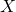and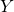be exponential random variables with parameters 1 and 2 respectively. Another random variableis defined as follows.

A coin, with probability p of Heads (and probability 1-p of Tails) is
tossed. DefinebyFind### Prerequisites

Cumulative Distribution Function

Exponential Distribution

## Solution :

Let ,be the CDF for i=X,Y, Z then we have ,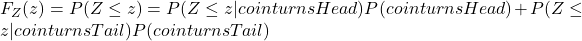=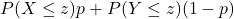=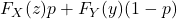Therefore pdf of Z is given by, where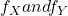are pdf of X,Y respectively .

So ,## Food For Thought

Find the the distribution function of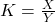and then find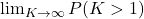## Subscribe to Cheenta at Youtube

This is a very beautiful sample problem from ISI MStat PSB 2008 Problem 7 based on finding the distribution of a random variable . Let's give it a try !!

## Problem- ISI MStat PSB 2008 Problem 7

Letandbe exponential random variables with parameters 1 and 2 respectively. Another random variableis defined as follows.

A coin, with probability p of Heads (and probability 1-p of Tails) is
tossed. DefinebyFind### Prerequisites

Cumulative Distribution Function

Exponential Distribution

## Solution :

Let ,be the CDF for i=X,Y, Z then we have ,==Therefore pdf of Z is given by, whereare pdf of X,Y respectively .

So ,## Food For Thought

Find the the distribution function ofand then find## Subscribe to Cheenta at Youtube

This site uses Akismet to reduce spam. Learn how your comment data is processed.

### Knowledge Partner